# Multivariate Kalman Filters¶

In :
#format the book
%matplotlib inline
from __future__ import division, print_function
import matplotlib.pyplot as plt
import book_format

Out:

## Introduction¶

The techniques in the last chapter are very powerful, but they only work in one dimension. The gaussians represent a mean and variance that are scalars - real numbers. They provide no way to represent multidimensional data, such as the position of a dog in a field. You may retort that you could use two Kalman filters for that case, one tracks the x coordinate and the other tracks the y coordinate. That does work in some cases, but put that thought aside, because soon you will see some enormous benefits to implementing the multidimensional case.

In this chapter I am purposefully glossing over many aspects of the mathematics behind Kalman filters. If you are familiar with the topic you will read statements that you disagree with because they contain simplifications that do not necessarily hold in more general cases. If you are not familiar with the topic, expect some paragraphs to be somewhat 'magical' - it will not be clear how I derived a certain result. I prefer that you develop an intuition for how these filters work through several worked examples. If I started by presenting a rigorous mathematical formulation you would be left scratching your head about what all these terms mean and how you might apply them to your problem. In later chapters I will provide a more rigorous mathematical foundation, and at that time I will have to either correct approximations that I made in this chapter or provide additional information that I did not cover here.

To make this possible we will restrict ourselves to a subset of problems which we can describe with Newton's equations of motion. In the literature these filters are called discretized continuous-time kinematic filters. In the next chapter we will develop the math required for solving any kind of dynamic system.

In this chapter we are dealing with a simpler form that we can discuss in terms of Newton's equations of motion: given a constant velocity v we can compute distance exactly with:

$$x = vt + x_0$$

If we instead assume constant acceleration we get

$$x = \frac{1}{2}at^2 + v_0 t + x_0$$

And if we assume constant jerk we get

$$x = \frac{1}{6}jt^3 + \frac{1}{2}a_0 t^2 + v_0 t + x_0$$

As a reminder, we can generate these equations using basic calculus. Given a constant velocity v we can compute the distance travelled over time with the equation

\begin{aligned} v &= \frac{dx}{dt}\\ dx &= v\, dt \\ \int_{x_0}^x\, dx &= \int_0^t v\, dt\\ x - x_0 &= vt - 0\\ x &= vt + x_0\end{aligned}

## Multivariate Normal Distributions¶

What might a multivariate normal distribution look like? In this context, multivariate just means multiple variables. Our goal is to be able to represent a normal distribution across multiple dimensions. Consider the 2 dimensional case. Let's say we believe that $x = 2$ and $y = 17$ This might be the x and y coordinates for the position of our dog, or the temperare and wind speed at our weather station, it doesn't really matter. We can see that for $N$ dimensions, we need $N$ means, like so:

$$\mu = \begin{bmatrix}{\mu}_1\\{\mu}_2\\ \vdots \\{\mu}_n\end{bmatrix}$$

Therefore for this example we would have

$$\mu = \begin{bmatrix}2\\17\end{bmatrix}$$

The next step is representing our variances. At first blush we might think we would also need N variances for N dimensions. We might want to say the variance for x is 10 and the variance for y is 4, like so.

$$\sigma^2 = \begin{bmatrix}10\\4\end{bmatrix}$$

This is incorrect because it does not consider the more general case. For example, suppose we were tracking house prices vs total $m^2$ of the floor plan. These numbers are correlated. It is not an exact correlation, but in general houses in the same neighborhood are more expensive if they have a larger floor plan. We want a way to express not only what we think the variance is in the price and the $m^2$, but also the degree to which they are correlated. It turns out that we use the following matrix to denote covariances with multivariate normal distributions. You might guess, correctly, that covariance is short for correlated variances.

$$\Sigma = \begin{bmatrix} \sigma_1^2 & \sigma_{12} & \cdots & \sigma_{1n} \\ \sigma_{21} &\sigma_2^2 & \cdots & \sigma_{2n} \\ \vdots & \vdots & \ddots & \vdots \\ \sigma_{n1} & \sigma_{n2} & \cdots & \sigma_n^2 \end{bmatrix}$$

If you haven't seen this before it is probably a bit confusing at the moment. Rather than explain the math right now, we will take our usual tactic of building our intuition first with various physical models. At this point, note that the diagonal contains the variance for each state variable, and that all off-diagonal elements (covariances) are represent how much the $i$th (row) and $j$th (column) state variable are linearly correlated to each other. In other words, it is a measure for how much they change together. No correlation will have a covariance of 0. So, for example, if the variance for x is 10, the variance for y is 4, and there is no linear correlation between x and y, then we would say

$$\Sigma = \begin{bmatrix}10&0\\0&4\end{bmatrix}$$

If there was a small amount of correlation between x and y we might have

$$\Sigma = \begin{bmatrix}10&1.2\\1.2&4\end{bmatrix}$$

where 1.2 is the covariance between x and y. Note that this is always symmetric - the covariance between x and y is always equal to the covariance between y and x. That is, $\sigma_{xy}=\sigma_{yx}$ for any x and y.

Now, without explanation, here is the full equation for the multivariate normal distribution in $n$ dimensions.

$$\mathcal{N}(\mu,\,\Sigma) = (2\pi)^{-\frac{n}{2}}|\Sigma|^{-\frac{1}{2}}\, e^{ -\frac{1}{2}(\mathbf{x}-\mu)^\mathsf{T}\Sigma^{-1}(\mathbf{x}-\mu) }$$

I urge you to not try to remember this function. We will program it in a Python function and then call it when we need to compute a specific value. However, if you look at it briefly you will note that it looks quite similar to the univariate normal distribution except it uses matrices instead of scalar values, and the root of $\pi$ is scaled by $n$. Here is the univariate equation for reference:

$$f(x, \mu, \sigma) = \frac{1}{\sigma\sqrt{2\pi}} e^{{-\frac{1}{2}}{(x-\mu)^2}/\sigma^2 }$$

If you are reasonably well-versed in linear algebra this equation should look quite manageable; if not, don't worry! Let's just plot it and see what it looks like.

In :
import mkf_internal
import numpy as np
mkf_internal.plot_3d_covariance((2, 17), np.array([[10., 0], [0, 4.]]))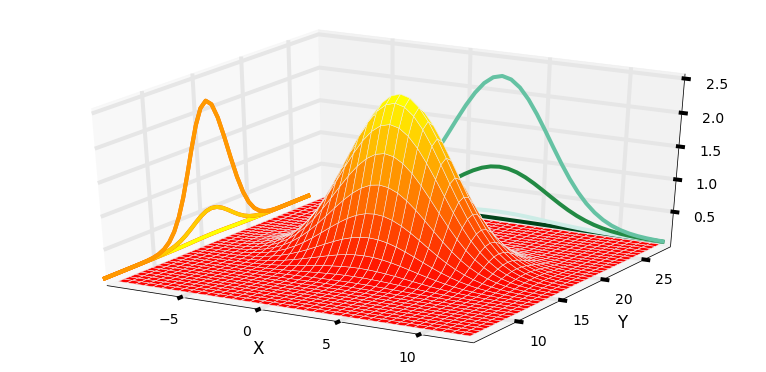Here we have plotted a two dimensional multivariate Gaussian with a mean of $\mu=[\begin{smallmatrix}2\\17\end{smallmatrix}]$ and a covariance of $\Sigma=[\begin{smallmatrix}10&0\\0&4\end{smallmatrix}]$. The three dimensional shape shows the probability of for any value of (x,y) in the z-axis. I have projected just the variance for x and y onto the walls of the chart - you can see that they take on the normal Gaussian bell curve shape. You can also see that, as we might hope, that the curve for x is wider than the curve for y, which is explained by $\sigma_x^2=10$ and $\sigma_y^2=4$. Also, the highest point of the curve is centered over (2,17), the means for x and y. I hope this demystifies the equation for you. Any multivariate Gaussian will create this sort of shape. If we think of this as a the Gaussian for our dog's position in a two dimensional field, the z-value at each point of (x,y) is the probability density for the dog being at that position. So, he has the highest probability of being near (2,17), a modest probability of being near (5,14), and a very low probability of being near (10,10).

We will discuss the mathematical description of covariances in the Kalman Filter math chapter. For this chapter we just need to understand the following.

1. The diagonal of the matrix contains the variance for each variable.

2. Each off-diagonal element contains $\sigma_{ij}$ - the covariance between i and j. This tells us how much linear correlation there is between the two variables. 0 means no correlation, and as the number gets higher the correlation gets greater.

3. $\sigma_{ij} = \sigma_{ji}$

4. The covariance between x and itself is just the variance of x: $\sigma_{xx} = \sigma_x^2$.

5. This chart only shows a 2 dimensional Gaussian, but the equation works for any number of dimensions >= 1. It's kind of hard to show a chart for the higher dimensions.

I have programmed the multivariate Gaussian equation and saved it in the file stats.py with the function name multivariate_gaussian. I am not showing the code here because I have taken advantage of the linear algebra solving apparatus of numpy to efficiently compute a solution - the code does not correspond to the equation in a one to one manner. If you wish to view the code, I urge you to either load it in an editor, or load it into this worksheet by putting %load -s multivariate_gaussian stats.py in the next cell and executing it with ctrl-enter.

However, please note that the Kalman filter equations incorporate this computation automatically; you will not be using this function very often in this book, so I would not spend a lot of time mastering this function unless it interests you.

As of version 0.14 scipy.stats has implemented the multivariate normal equation with the function multivariate_normal(). It implements a 'frozen' form where you set the mean and covariance once, and then calculate the probability for any number of values for x over any arbitrary number of calls. This is much more efficient then recomputing everything in each call. So, if you have version 0.14 or later you may want to substitute my function for the built in version. Use scipy.version.version to get the version number. I deliberately named my function multivariate_gaussian() to ensure it is never confused with the built in version. I will say that for a single call, where the frozen variables do not matter, mine consistently runs faster as measured by the timeit function.

The tutorial for the scipy.stats module explains 'freezing' distributions and other very useful features. As of this date, it includes an example of using the multivariate_normal function, which does work a bit differently from my function.

In :
from stats import gaussian, multivariate_gaussian


Let's use it to compute a few values just to make sure we know how to call and use the function, and then move on to more interesting things.

First, let's find the probability density for our dog being at (2.5, 7.3) if we believe he is at (2, 7) with a variance of 8 for $x$ and a variance of 4 for $y$.

Start by setting $x$ to (2.5, 7.3):

In :
x = [2.5, 7.3]


Next, we set the mean of our belief:

In :
mu = [2.0, 7.0]


Finally, we have to define our covariance matrix. In the problem statement we did not mention any correlation between $x$ and $y$, and we will assume there is none. This makes sense; a dog can choose to independently wander in either the $x$ direction or $y$ direction without affecting the other. If there is no correlation between the values you just fill in the diagonal of the covariance matrix with the variances. I will use the seemingly arbitrary name $\textbf{P}$ for the covariance matrix. The Kalman filters use the name $\textbf{P}$ for this matrix, so I will introduce the terminology now to avoid explaining why I change the name later.

In :
P = [[8.,  0.],
[0., 4.]]


Now just call the function

In :
print('{:.4}'.format(multivariate_gaussian(x, mu, P)))

0.02739


Note that the function can accept lists, np.array, or np.matrix as arguments, or even scalars. Type multivariate_gaussian? in a cell and press ctrl-enter to get the help for this function.

These numbers are not easy to interpret. Let's plot this in 3D, with the $z$ (up) coordinate being the probability distribution.

In :
import mkf_internal
mkf_internal.plot_3d_covariance(mu, P)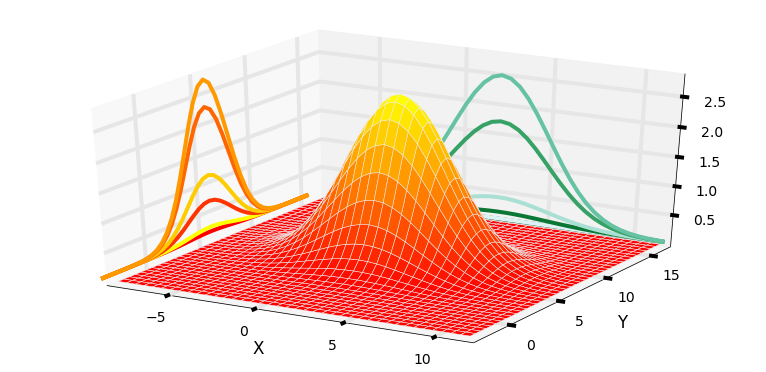The result is clearly a 3D bell shaped curve. We can see that the gaussian is centered around (2,7), and that the probability density quickly drops away in all directions. On the sides of the plot I have drawn the Gaussians for $x$ in greens and for $y$ in orange.

Let's look at this in a slightly different way. Instead of plotting a surface showing the probability distribution I will just generate 1,000 points with the distribution of $[\begin{smallmatrix}8&0\\0&4\end{smallmatrix}]$.

In :
mkf_internal.plot_3d_sampled_covariance(mu, P)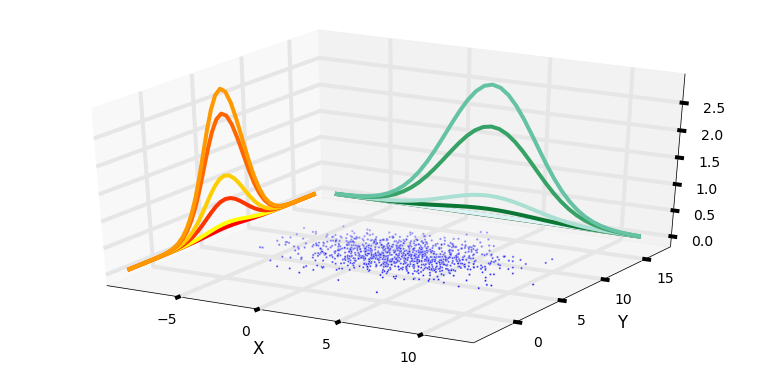We can think of the sampled points as being possible locations for our dog given those particular mean and covariances. The contours on the side show the variance in the points for $x$ and $y$ only.

As beautiful as this is, it is perhaps a bit hard to get useful information. For example, it is not easy to tell if $x$ and $y$ both have the same variance or not. So for most of the rest of this book we will display multidimensional Gaussian using contour plots. I will use some helper functions in stats.py to plot them. If you are interested in linear algebra go ahead and look at the code used to produce these contours, otherwise feel free to ignore it.

In :
import stats

P = np.array([[2, 0], [0, 2]])
plt.subplot(131)
stats.plot_covariance_ellipse((2, 7), cov=P, facecolor='g', alpha=0.2,
title='|2 0|\n|0 2|', axis_equal=False)
plt.ylim((4, 10))

plt.subplot(132)
P = np.array([[2, 0], [0, 9]])
plt.ylim((4, 10))
stats.plot_covariance_ellipse((2, 7), P,  facecolor='g', alpha=0.2,
axis_equal=False, title='|2 0|\n|0 9|')

plt.subplot(133)
P = np.array([[2, 1.2], [1.2, 2]])
plt.ylim((4, 10))
stats.plot_covariance_ellipse((2, 7), P,  facecolor='g', alpha=0.2,
axis_equal=False,
title='|2 1.2|\n|1.2 2|')

plt.tight_layout()
plt.show()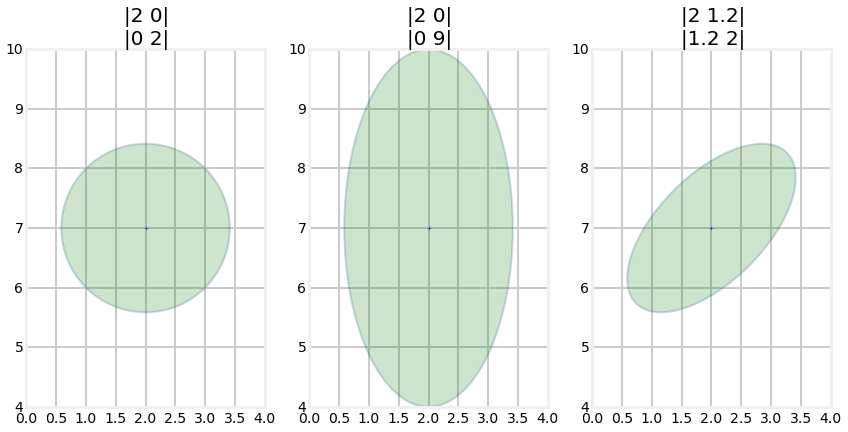And for those of you viewing this online or in IPython Notebook on your computer, here is an animation.From a mathematical perspective these display the values that the multivariate gaussian takes for a specific standard deviation. This is like taking a horizontal slice out of the 3D plot. By default it displays one standard deviation, but you can use the variance parameter to control what is displayed. For example, variance=3**2 would display the 3rd standard deviation, and variance=[1,4,9] would display the 1st, 2nd, and 3rd standard deviations as in the chart below. This takes 3 different horizontal slices of the multivariate Gaussian chart and displays them in 2D.

In :
P = np.array([[2, 0], [0, 9]])

stats.plot_covariance_ellipse((2, 7), P,  facecolor='g', alpha=0.2,
variance=[1, 2**2, 3**2],
axis_equal=True, title='|2 0|\n|0 9|')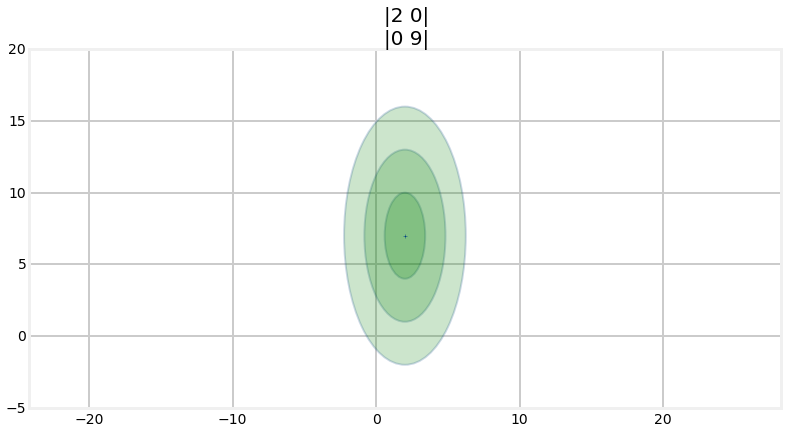Thinking about the physical interpretation of these plots clarifies their meaning. The first plot uses the mean and covariance matrices of \begin{aligned} \mathbf{\mu} &= \begin{bmatrix}2\\7\end{bmatrix} \\ \Sigma &= \begin{bmatrix}2&0\\0&2\end{bmatrix} \end{aligned}

Let this be our current belief about the position of our dog in a field. In other words, we believe that he is positioned at (2,7) with a variance of $\sigma^2=2$ for both x and y. The contour plot shows where we believe the dog is located with the '+' in the center of the ellipse. The ellipse shows the boundary for the $1\sigma$ probability, where $1\sigma$ is one standard deviation. In other words, for this Gaussian 68% of the data will fall within this ellipse. Recall from the Gaussians chapter the the 68-95-99.7 rule - 68% of all values will fall within 1 standard deviation ($1\sigma$), 95% within $2\sigma$, and 99.7% within $3\sigma$. The dog could be at (356443,58483), but the chances for values that far away from the mean are infitestimally small.

An equivalent way of thinking about this is the circle/ellipse shows us the amount of error in our belief. A tiny circle would indicate that we have a very small error, and a very large circle indicates a lot of error in our belief. We will use this throughout the rest of the book to display and evaluate the accuracy of our filters at any point in time.

The second plot uses the mean and covariance matrices of

\begin{aligned} \mu &=\begin{bmatrix}2\\7\end{bmatrix} \\ \Sigma &= \begin{bmatrix}2&0\\0&9\end{bmatrix} \end{aligned}

This time we use a different variance for $x$ (2) vs $y$ (9). The result is an ellipse. When we look at it we can immediately tell that we have a lot more uncertainty in the $y$ value vs the $x$ value. Our belief that the value is (2,7) is the same in both cases, but errors are different. In this case the standard deviation in $x$ is $\sigma_x = \sqrt{2}=1.414$ and the standard deviation for $y$ is $\sigma_y = \sqrt{9}=3$. This sort of thing happens naturally as we track objects in the world - one sensor has a better view of the object, or is closer, than another sensor, and so we end up with different error rates in the different axis.

The third plot uses the mean and covariance matrices of: \begin{aligned} \mu &=\begin{bmatrix}2\\7\end{bmatrix} \\ \Sigma &= \begin{bmatrix}2&1.2\\1.2&2\end{bmatrix} \end{aligned}

This is the first contour that has values in the off-diagonal elements of $cov$, and this is the first contour plot with a slanted ellipse. This is not a coincidence. The two facts are telling use the same thing. A slanted ellipse tells us that the $x$ and $y$ values are somehow correlated. We denote that in the covariance matrix with values off the diagonal. What does this mean in physical terms? Think of trying to park your car in a parking spot. You can not pull up beside the spot and then move sideways into the space because most cars cannot go purely sideways. $x$ and $y$ are not independent. This is a consequence of the steering system in a car. When your tires are turned the car rotates around its rear axle while moving forward. Or think of a horse attached to a pivoting exercise bar in a corral. The horse can only walk in circles, he cannot vary $x$ and $y$ independently, which means he cannot walk straight forward to to the side. If $x$ changes, $y$ must also change in a defined way.

So when we see this ellipse we know that $x$ and $y$ are correlated, and that the correlation is "strong". The size of the ellipse shows how much error we have in each axis, and the slant shows how strongly correlated the values are.

Let's look at this in a graph and then reason about it. Let's assume that we have a covariance for the position of our dog, which we believe is at coordinate (5, 10) meters. If the standard deviation in x and y is each 2 meters, but they are strongly correlated, our covariance contour would look like this.

In :
P = np.array([[4, 3.9], [3.9, 4]])
stats.plot_covariance_ellipse((5, 10), P, edgecolor='k',
variance=[1, 2**2, 3**2])
plt.xlabel('X')
plt.ylabel('Y')
plt.show()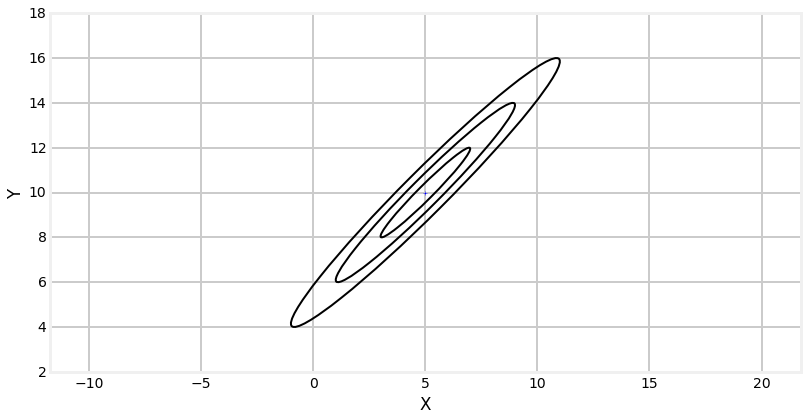Now suppose I were to tell you that the actual position of the dog in the x dimension is 7.5, what can we infer about his position in y? The position is extremely likely to lie within the 3$\sigma$ covariance, as in the chart below. We can infer the like position in y based on the covariance matrix because there is a correlation between x and y.

In :
P = np.array([[4, 3.9], [3.9, 4]])
stats.plot_covariance_ellipse((5, 10), P, edgecolor='k',
variance=[1, 2**2, 3**2])
plt.xlabel('X')
plt.ylabel('Y')
plt.gca().autoscale(tight=True)
plt.axvline(7.5, ls='--', lw=1)
plt.axhline(12.5, ls='--', lw=1)
plt.scatter(7.5, 12.5, s=2000, alpha=0.5)
plt.plot()
plt.show()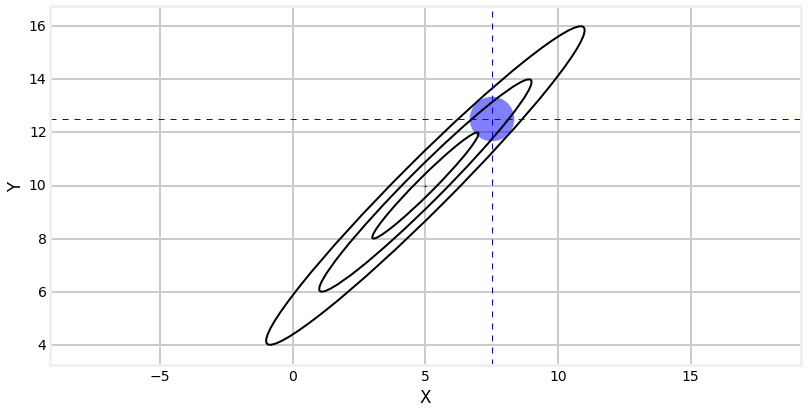A word about correlation and independence. If variables are independent they can vary separately. If you walk in an open field, you can move in the $x$ direction (east-west), the $y$ direction(north-south), or any combination thereof. Independent variables are always also uncorrelated. Except in special cases, the reverse does not hold true. Variables can be uncorrelated, but dependent. For example, consider the pair$(x,y)$ where $y=x^2$. Correlation is a linear measurement, so $x$ and $y$ are uncorrelated. However, they are obviously dependent on each other.

## Multiplying Multidemensional Gaussians¶

In the previous chapter we incorporated an uncertain measurement with an uncertain estimate by multipling their Gaussians together. The result was another Gaussian with a smaller variance. If two piece of uncertain information corroborate each other we should be more certain in our conclusion. The graphs look like this:

In :
import stats
xs = np.arange(-5, 10, 0.1)

mean1, var1 = 0, 5
mean2, var2 = 5, 1
mean, var = stats.mul(mean1, var1, mean2, var2)

ys = [stats.gaussian(x, mean1, var1) for x in xs]
plt.plot(xs, ys, label='M1')

ys = [stats.gaussian(x, mean2, var2) for x in xs]
plt.plot(xs, ys, label='M2')

ys = [stats.gaussian(x, mean, var) for x in xs]
plt.plot(xs, ys, label='M1 x M2')
plt.legend()
plt.show()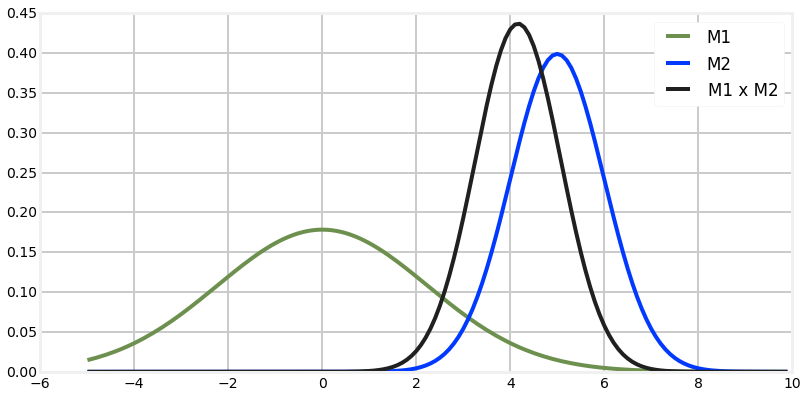The combination of measurement 1 and 2 yields more certainty, so the new Gaussian is taller and narrower - the variance became smaller. The same thing happens in multiple dimensions with multivariate Gaussians.

Here are the equations for multiplying multivariate Gaussians. You will not need to remember these equations, as they are backed into the Kalman filter equations that will be presented shortly. This computation is also available in FilterPy using the multivariate_multiply() method, which you can import from filterpy.common.

\begin{aligned} \mu &= \Sigma_2(\Sigma_1 + \Sigma_2)^{-1}\mu_1 + \Sigma_1(\Sigma_1 + \Sigma_2)^{-1}\mu_2 \\ \Sigma &= \Sigma_1(\Sigma_1+\Sigma_2)^{-1}\Sigma_2 \end{aligned}

\begin{aligned} \mu &=\frac{\sigma_1^2 \mu_2 + \sigma_2^2 \mu_1} {\sigma_1^2 + \sigma_2^2}, \\ \sigma^2 &= \frac{1}{\frac{1}{\sigma_1^2} + \frac{1}{\sigma_2^2}} \end{aligned}

This looks similar to the equations for the multivariate equations. This will be more obvious if you recognize that matrix inversion, denoted by the -1 power, is like division since $AA^{-1} =I$. I will rewrite the inversions as divisions - this is not a mathematically correct thing to do as the order of operations becomes ambiguous (matrix multiplication is not commutative), but it does help us see what is going on.

\begin{aligned}\mu &\approx \frac{\Sigma_2\mu_1 + \Sigma_1\mu_2}{\Sigma_1 + \Sigma_2} \\ \Sigma &\approx \frac{\Sigma_1\Sigma_2}{(\Sigma_1+\Sigma_2)} \end{aligned}

In this form we can see that these equations are just the linear algebra form of the univariate equations.

Now Let's explore multivariate Gaussians in terms of a concrete example. Suppose that we are tracking an aircraft with a couple of radar systems. I will ignore altitude as this is easier to graph in two dimensions. Radars give us the range and bearing to a target. We start out being uncertain about the position of the aircraft, so the covariance, which is just our uncertainty about the position, might look like this.

In :
P0 = np.array([[6, 0], [0, 6]])
stats.plot_covariance_ellipse((10, 10), P0, facecolor='y', alpha=0.6)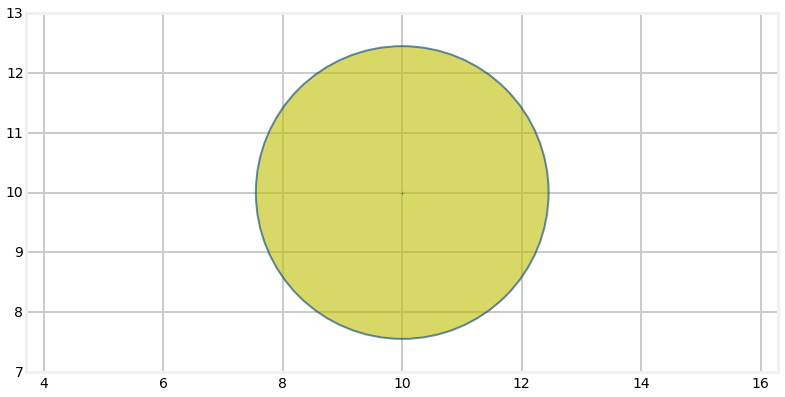Now suppose that there is a radar to the lower right of the aircraft. Further suppose that the radar is very accurate in the bearing measurement, but not very accurate at the range. That covariance, which is just the uncertainty in the reading might look like this (plotted in blue):

In :
P1 = np.array([[2, 1.9], [1.9, 2]])
stats.plot_covariance_ellipse((10, 10), P0, facecolor='y', alpha=0.6)
stats.plot_covariance_ellipse((10, 10), P1, facecolor='b', alpha=0.6)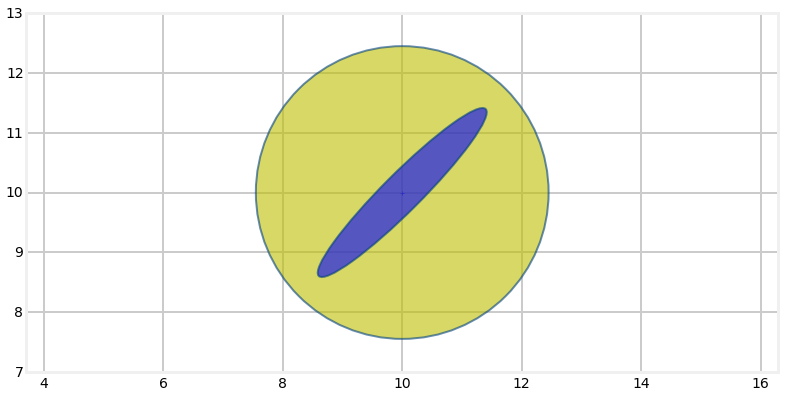To combine the information we multiply the two Gaussians together. Let's see the result of that. I will use FilterPy's multivarite_multiply method to perform the multiplication.

In :
from filterpy.common import multivariate_multiply

P2 = multivariate_multiply((10,10), P0, (10,10), P1)

stats.plot_covariance_ellipse((10, 10), P0, facecolor='y', alpha=0.2)
stats.plot_covariance_ellipse((10, 10), P1, facecolor='b', alpha=0.6)
stats.plot_covariance_ellipse((10, 10), P2, facecolor='y')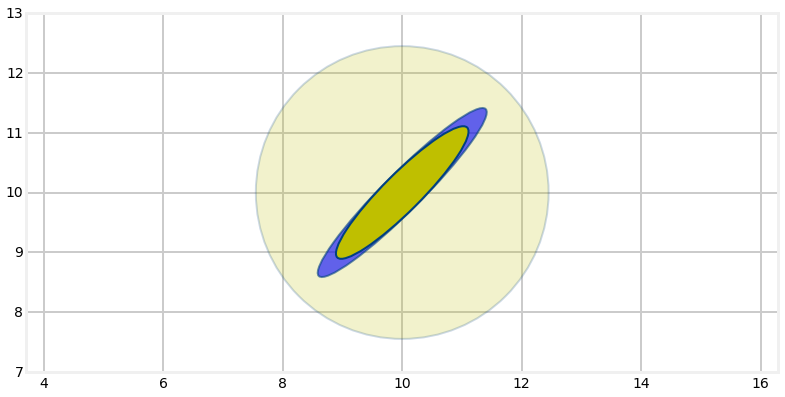Here I have plotted the orignal estimate it a very transparent yellow, the radar reading in blue, and the estimate generate by multiplying the two Gaussians together in yellow.

It retained the same shape and position as the radar measurement, but is smaller. We've seen this with the one dimensional Gaussians - multiplying two Gaussians makes the variance smaller because we are incorporating more information, hence we are less uncertain. But the main point I want to make is that the covariance shape reflects the physical layout of the aircraft and the radar system.

Now lets say we get a measurement from a second radar bank, this one to the lower right, which I will plot in blue against the yellow covariance of our current belief.

In :
P3 = np.array([[2, -1.9], [-1.9, 2.2]])
stats.plot_covariance_ellipse((10, 10), P2, facecolor='y', alpha=0.6)
stats.plot_covariance_ellipse((10, 10), P3, facecolor='b', alpha=0.6)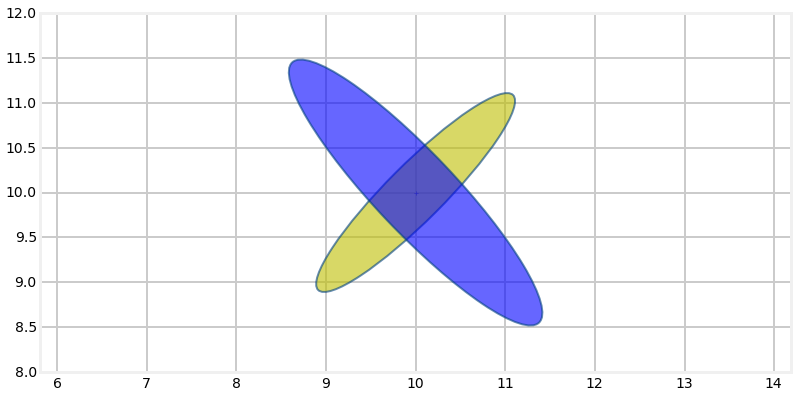Again, to incorprate this new information we will multiply the Gaussians together.

In :
P4 = multivariate_multiply((10,10), P2, (10,10), P3)

stats.plot_covariance_ellipse((10, 10), P2, facecolor='y', alpha=0.2)
stats.plot_covariance_ellipse((10, 10), P3, facecolor='b', alpha=0.6)
stats.plot_covariance_ellipse((10, 10), P4, facecolor='y')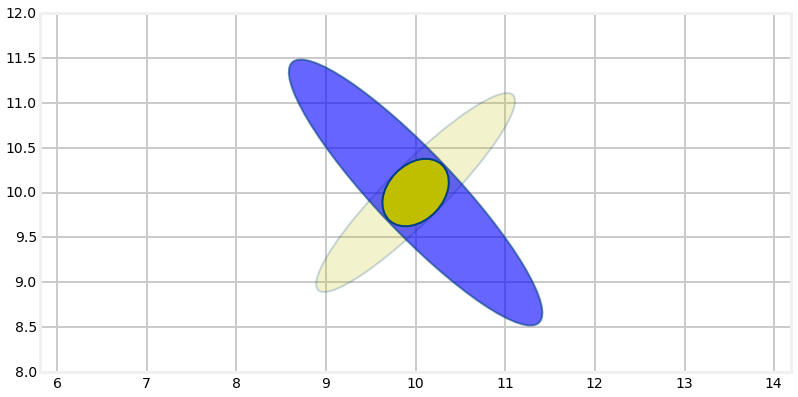You can see how the multivariate Gaussian's shape reflects the geometry of the problem. The first radar system was at a 45 degree angle to the aircraft, and its error in the bearing measurement was much smaller than the error in the range. This resulted in a long and narrow covariance ellipse whose major axis was aligned with the angle to the radar system. The next radar system was also at a 45 degree angle, but to the right, so the two measurements were orthogonal to each other. This allowed us to triangulate on the aircraft, which resulted in a very accurate final estimate. We didn't explicitly write any code to perform triangulation; it was a natural outcome of multiplying the Gaussians of each measurement together.

To make sure you understand this, what would the Gaussian look like if we only had one radar station, and we receive several measurements from it over a short period of time? Clearly the Gaussian would remain elongated in the axis of the bearing angle. Without a second radar station no information would be provided to reduce the error on that axis, so it would remain quite large. As the aircraft moves the bearing will typically change by a small amount, so over time some of the error will be reduced, but it will never be reduced as much as a second radar station would provide.

To round this out lets quickly redo this example but with the first radar system in a different position. I will position it directly to the left of the aircraft. The only change I need to make is to the Gaussian for the measurement from the radar. I used

$$\Sigma = \begin{bmatrix}2&1.9\\1.9&2\end{bmatrix}$$

Why did this result in a 45 degree ellipse? Think about that before reading on. It was 45 degrees because the values in the diagonal were identical. So if x=10 then y=10, and so on. We can alter the angle by making the variance for x or y different, like so:

In :
P1 = np.array([[2, 1.9], [1.9, 8]])
stats.plot_covariance_ellipse((10, 10), P1, facecolor='y', alpha=0.6)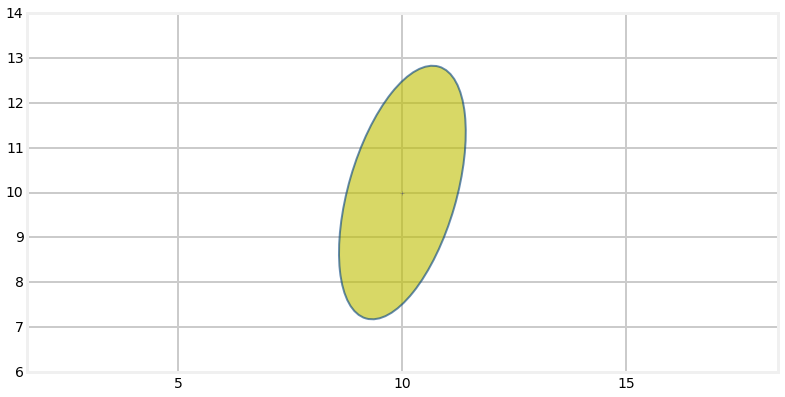The radar is to the left of the aircraft, so I can use a covariance of $$\Sigma = \begin{bmatrix}2&1.9\\1.9&2\end{bmatrix}$$ to model the measurement. In the next graph I plot the original estimate in a very light yellow, the radar measurement in blue, and the new estimate based on multiplying the two Gaussians together in yellow.

In :
P1 = np.array([[2, 0], [0, .2]])

P2 = multivariate_multiply((10,10), P0, (10,10), P1)

stats.plot_covariance_ellipse((10, 10), P0, facecolor='y', alpha=0.2)
stats.plot_covariance_ellipse((10, 10), P1, facecolor='b', alpha=0.6)
stats.plot_covariance_ellipse((10, 10), P2, facecolor='y')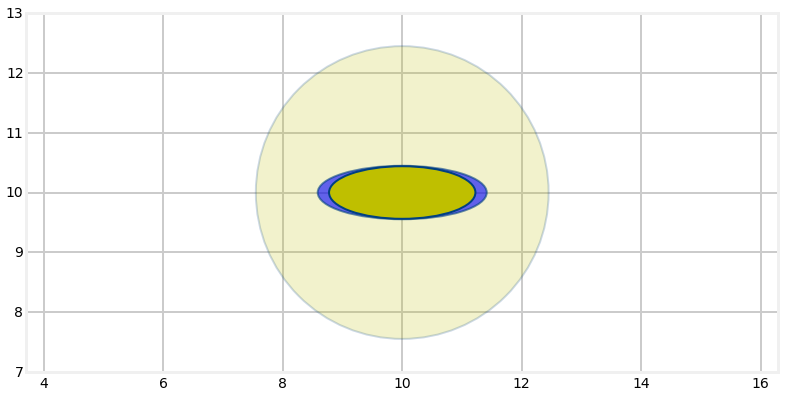Now we can incorporate the measurement from the second radar system, which we will leave in the same position as before.

In :
P3 = np.array([[2, -1.9], [-1.9, 2.2]])
P4 = multivariate_multiply((10,10), P2, (10,10), P3)

stats.plot_covariance_ellipse((10, 10), P2, facecolor='y', alpha=0.2)
stats.plot_covariance_ellipse((10, 10), P3, facecolor='b', alpha=0.6)
stats.plot_covariance_ellipse((10, 10), P4, facecolor='y')